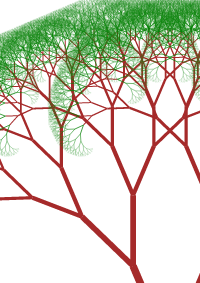# Create Binary Trees using Javascript and HTML5 Canvas

View code Play Walkthrough### Description

Create random binary(fractal) trees using Javascript and HTML5 canvas.

The trees are created using simple lines drawn recursively on the canvas. Every 500ms(0.5 seconds) a new random tree is generated.

A bit of math is involved as simple vectors have been used in this example for calculating branch points.(close)#### MOHSIN

Suggestion:#### MOHSIN

A simple implementation, . function drawTree(x1,y1,length,angle,n){
var x2=x1+length*Math.cos(angle*Math.PI/180);//new x2
var y2=y1-length*Math.sin(angle*Math.PI/180);//new y2
ctx.beginPath(); //
ctx.moveTo(x1,y1); // the drawing part
ctx.lineTo(x2,y2); //
if(n&gt;2)
ctx.strokeStyle=”brown”;// line color near base of tree
else
ctx.strokeStyle=”green”;// line color at leaves
ctx.lineWidth=n-1;//decreasing line-width as tree gets extended
ctx.stroke();// paint the tree
if(n&gt;0)// recursion
{
drawTree(x2,y2,length*0.75,angle+40,n-1);
drawTree(x2,y2,length*0.75,angle-40,n-1);
}
}#### AlexWindHope

why fillRect? clearRect seems to b fine here#### kcy1019

So nice tutorial!
Thanks :)

5x 10x 15x 20x